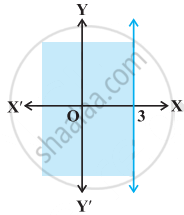# Graph of x < 3 is - Mathematics

MCQ
True or False

Graph of x < 3 is• True

• False

#### Solution

This statement is False.

Explanation:

The given graph represents x ≤ 3

Concept: Graphical Solution of Linear Inequalities in Two Variables
Is there an error in this question or solution?

#### APPEARS IN

NCERT Mathematics Exemplar Class 11
Chapter 6 Linear Inequalities
Exercise | Q 31.(x) | Page 111

Share